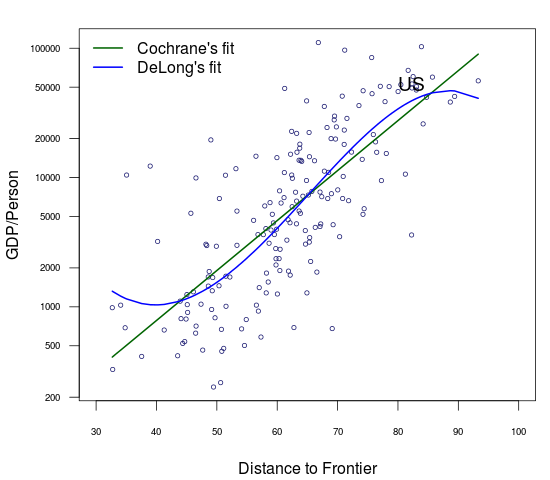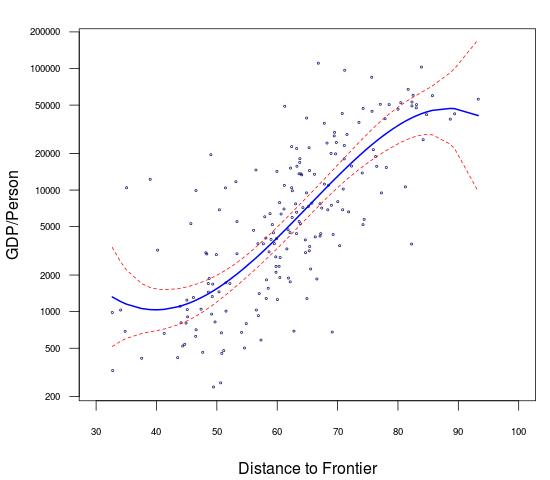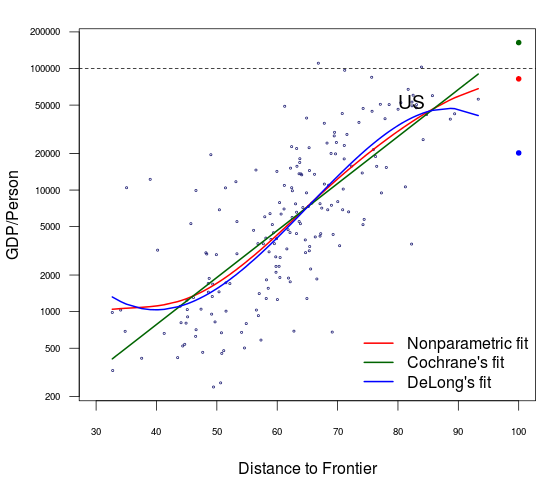## Monday, July 11, 2016

### The gains to US GDP from a Doing Business score of 100

by Dhananjay Ghei and Nikita Singh.

Can a country achieve growth by implementing large pro-business reforms? If yes, then how much growth is really possible from such reforms? In a recent WSJ op-ed, Cochrane takes a stab at this question for the United States. Using data from the World Bank's ease of doing business index, Cochrane claims there is a log-linear relationship between GDP per person and business climate. By extrapolating this relationship out of sample, he predicts that the US would register a 209% improvement in per capita income (or, 6% additional annual growth if the required reforms are implemented over the next 20 years) by achieving the ease of doing business index value of 100.

Brad Delong disagrees. He fits a fourth-degree polynomial on the same data. He justifies this on the grounds that the third degree coefficient is negative and statistically significant. His forecast shows that an increase in the index value beyond 90 would actually lead to a lower GDP per person. Figure 1 juxtaposes the log-linear and polynomial regression fit, and we can see how the two views are sharply different. The straight line yields higher and higher GDP as you go to 100; the polynomial droops off at the end.Figure 1: The analysis of John Cochrane and Brad DeLong

### Areas of concern

There are many areas of concern with this analysis:

1. Assuming linearity is surely a stretch. But polynomial regressions are a bad way to deal with nonlinearity. In particular, polynomial regressions are very fragile at the end points. This can be easily seen in Figure 2 as the prediction interval increases at edges of the data. In addition, extrapolation using a polynomial is almost always sure to give a wrong answer as the curvature of the polynomial is unidentified outside the sample.
2. Using a cross-sectional regression with one variable is a poor guide to the causal relationships. Labour and capital matter to GDP per capita. There are stark differences in law and governance, institutions and culture across countries; it is unlikely that the doing business score is a sufficient statistic.
3. Hallward-Driemeier and Pritchett (2015) show that the "doing business index" is not a good reflection of how the laws on paper are implemented in reality. The main point of their argument is that better de jure regulations do not necessarily imply improved de facto outcomes specially when a country has weak governmental capabilities for implementation and enforcement. Even if the US does well on the rule of law, and this gap between rules and deals is absent, this is a serious issue for many (most?) observations in the dataset.Figure 2: The 95% prediction interval for the polynomial regression

### Can we do better?

Criticisms 2 and 3 are hard to handle. But a little bit of statistics helps us do better on the first. We use non-parametric regression as a way to have nonlinearity in the relationship between business climate and GDP per person without having to take a stand on a particular functional form. This involves three steps:

1. Selecting an optimum bandwidth using cross-validation
2. Estimating a nonparametric model using the chosen bandwidth
3. Tests of statistical significance and specification

We use a second order Gaussian kernel and fit a local linear estimator to identify the functional form in sample. Business climate is significant at 1% level in the local linear non-parametric model. Moreover, based on a lower cross validation score, the non-parametric regression is favoured.  In addition, we do a bunch of robustness tests by changing the type of kernel and regression. The results do not change much in either of the cases. These calculations were done in  R using the np package.Figure 3: Non-parametric regression gives us the best of both worlds

The results, shown above, show that there is nonlinearity in the data. The linear model used by Cochrane is not appropriate. But we're better off as compared with using a polynomial regression; the confidence interval is tighter at the edges.

Figure 4 superposes the three models. The coloured dots show the predicted value of GDP per person using the three different specifications when the doing business index takes the value of 100.Figure 4: Comparing the three predictions

Our nonparametric estimate shows that gains from achieving a score beyond 90 are increasing and somewhere in between Cochrane and DeLong's numbers. Cochrane predicts that the US would achieve 6% additional annual growth for 20 years by moving to a score of 100. If we go out of sample to estimate using the nonparametric fit, this shows an annual growth of 2.22% for the next 20 years. This is not something to laugh at, but it's a smaller, and we think a more plausible estimate.

### References

Hallward-Driemeier, Mary and Lant Pritchett. 2015. "How Business Is Done in the Developing World: Deals versus Rules." Journal of Economic Perspectives, 29(3): 121-40.

Tristen Hayfield and Jeffrey S. Racine (2008). Nonparametric Econometrics: The np Package. Journal of Statistical Software 27(5). URL http://www.jstatsoft.org/v27/i05/.

Dhananjay Ghei is a researcher at the National Institute of Public Finance and Policy. Nikita Singh is a MRes. student at London School of Economics and Political Science. The authors thank Ajay Shah for valuable discussions and feedback.

LaTeX mathematics works. This means that if you want to say $10 you have to say \$10.